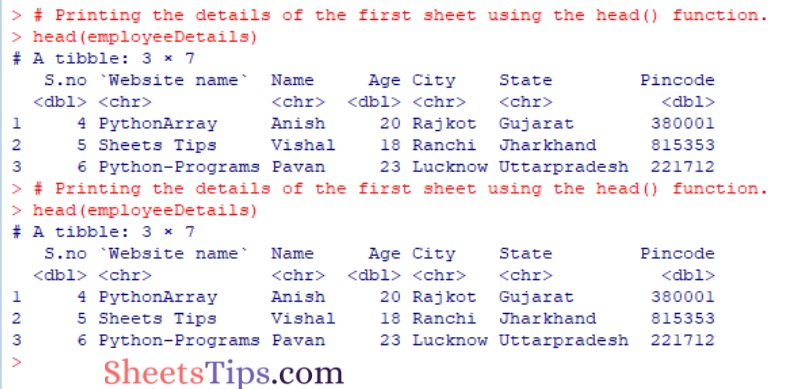# R Program to Import Multiple Excel Sheets

Usually, we work with several data sources, and sometimes we come across tables spread across multiple Excel pages. With the help of the read excel() function in R language, one can easily import the sheets. However, the read_excel() function imports the first sheet by default. Thus, it is important to specify the number or name of the argument sheet in order to import a different sheet. Let us discuss how to specify the number or name of the argument sheet to import multiple Excel sheets into R Language using examples in this article. Read on to find out more.

## read_excel Package in R to Import Excel Worksheets

The readxl package in R is used to import and read Excel workbooks, allowing you to quickly work with and modify.xslsx sheets. The following syntax can be used to install and load it into the R working environment.

Recommended Reading On: Java Program to Print Series 5 25 125 625 3125…N

Installation:

```install.packages("readxl")

```

Output:

```Installing package into ‘C:/Users/cirus/AppData/Local/R/win-library/4.2’
(as ‘lib’ is unspecified)
--- Please select a CRAN mirror for use in this session ---
Content type 'application/zip' length 1197654 bytes (1.1 MB)

package ‘readxl’ successfully unpacked and MD5 sums checked

## Program to Import Multiple Excel Sheets in R

Functions:

The FUNCTION here is the read_excel method of this package store, which reads the contents of the specified sheet name into a tibble, which is a tabular-like structure used to store data in fixed rows and columns.

The read_excel method is applied to all sheets of the workbook by the lapply() method.

Syntax:

`read_excel(path, sheet)`

Parameters

path: It is the path of the file given as input.

sheet: It is the sheet name to be read.

Approach:

• Importing readxl package using the library() function.
• Import the excel workbook for checking the number of worksheets it contains by passing the path of the excel file as an argument to the excel_sheets() function.

Below is the Implementation:

```# Importing readxl package using the library() function
# Import the excel workbook for checking the number of worksheets it contains
# by passing path of excel file as an argument to the excel_sheets() function.

Output:

` "First Batch " "Second Batch"`

### Step #2:  Importing Multiple Excel Sheets

Approach:

• Import the Sheets using the read_excel() function by passing the excel path and sheet name as arguments to it.
• Printing the details of the first sheet using the head() function.
• Printing the details of the first sheet using the head() function.

Below is the Implementation:

```# Import the Sheets using the read_excel() function by passing excel path and sheet name as arguments to it.
sheet = 1)
sheet = "First Batch ")
sheet = "Second Batch")

# Printing the details of the first sheet using the head() function.
# Printing the details of the first sheet using the head() function.
```

Output:### Step #3: Full Code

Approach:

• Importing readxl package using the library() function.
• Import the excel workbook for checking the number of worksheets it contains by passing the path of the excel file as an argument to the excel_sheets() function.
• Import the Sheets using the read_excel() function by passing the excel path and sheet name as arguments to it.
• Printing the details of the first sheet using the head() function.
• Printing the details of the first sheet using the head() function.
• The Exit of the Program.

Below is the Implementation:

```# Importing readxl package using the library() function
# Import the excel workbook for checking the number of worksheets it contains
# by passing path of excel file as an argument to the excel_sheets() function.
excel_sheets("StudentData.xlsx")
# Import the Sheets using the read_excel() function by passing excel path and sheet name as arguments to it.
sheet = 1)
sheet = "First Batch ")